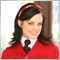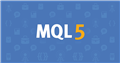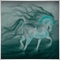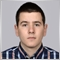# Calculate profit in dollar20

Hello,

I am  new in MQL coding, I am trying to calculate profit from an open price (set in an input variable), the code is below.

The problem is OrderProfit() is returning a different value with my code.

===================

//+------------------------------------------------------------------+
//| Expert initialization function                                   |
//+------------------------------------------------------------------+
input string Pair1 = "GBPJPY"; // Pair 1
input double Price1 = 132.800;
input double lot1 = 0.01;

void OnTick()
{
//---

//cek_Gap(Pair1, Pair2, Price1, Price2);
double var1 = Price1 ;

double vbid1    = MarketInfo(Pair1,MODE_BID);
double vpoint1  = MarketInfo(Pair1,MODE_POINT);
int    vdigits1 = MarketInfo(Pair1,MODE_DIGITS);
int   pip1;

string mktInfo1, mktInfo2, mktInfo1a, mktInfo2a;
mktInfo1  = Pair1 +" > BID: " +vbid1 +"; ASK: " +vask1  +"; DIGITS: " +vdigits1 +"; SPREAD: " +vspread1 +"; POINT: " +NormalizeDouble(vpoint1,vdigits1);
mktInfo1a = Pair1 +" > POINT: " +DoubleToStr(vpoint1,vdigits1);

pips1 = MathAbs(vbid-var1)/Point;
} else {
}
//Print(DiffPips);

double DiffPips1 = (NormalizeDouble(vbid1-var1,vdigits1)/vpoint1) * lot1;

Print(DiffPips1);

}

===================

Please ignore the unecessary code, I am  trying to lear MQL get some value from bid, ask, digit, spread, etc..

P.S. I am trying to follow the mql5.com rule, by putting the code in block code, but not shown so i put manual like above..Documentation on MQL5: Trade Functions / OrderCalcProfit
• www.mql5.com
The function calculates the profit for the current account, in the current market conditions, based on the parameters passed. The function is used for pre-evaluation of the result of a trade operation. The value is returned in the account currency. [out]  The variable, to which the calculated value of the profit will be written in case the...6212

Where is order profit in your code7175

Hey,

I think when you calculate DiffPips you need to account for the TickValue as well in this calculation

`   double DiffPips1 = (NormalizeDouble(vbid1-var1,vdigits1)/vpoint1) * lot1;`

And one question - is this your way to calculate the currency profit ? or the pip difference ?20

Ahmet Metin Yilmaz:
Where is order profit in your code

I don't put it on the code, I just compared it with the order profit in trade tab.

but good idea, I will print it too..

thanks20

Stanislav Ivanov:

Hey,

I think when you calculate DiffPips you need to account for the TickValue as well in this calculation

And one question - is this your way to calculate the currency profit ? or the pip difference ?

Hi Stanis,

not sure what you mean, can you give an example of this ThickValue ?

thanks22709

Pene Jumawad: give an example of this ThickValue ?

Risk depends on your initial stop loss, lot size, and the value of the symbol. It does not depend on margin and leverage. No SL means you have infinite risk. Never risk more than a small percentage of your trading funds, certainly less than 2% per trade, 6% total.

1. You place the stop where it needs to be — where the reason for the trade is no longer valid. E.g. trading a support bounce the stop goes below the support.

2. AccountBalance * percent/100 = RISK = OrderLots * (|OrderOpenPrice - OrderStopLoss| * DeltaPerLot + CommissionPerLot) (Note OOP-OSL includes the spread, and DeltaPerLot is usually around \$10/pip but it takes account of the exchange rates of the pair vs. your account currency.)

3. Do NOT use TickValue by itself - DeltaPerLot and verify that MODE_TICKVALUE is returning a value in your deposit currency, as promised by the documentation, or whether it is returning a value in the instrument's base currency.
MODE_TICKVALUE is not reliable on non-fx instruments with many brokers - MQL4 programming forum 2017.10.10
Is there an universal solution for Tick value? - Currency Pairs - General - MQL5 programming forum 2018.02.11
Lot value calculation off by a factor of 100 - MQL5 programming forum 2019.07.19

4. You must normalize lots properly and check against min and max.

5. You must also check FreeMargin to avoid stop out

Most pairs are worth about \$10 per PIP. A \$5 risk with a (very small) 5 PIP SL is \$5/\$10/5 or 0.1 Lots maximum.20

William Roeder:

Risk depends on your initial stop loss, lot size, and the value of the symbol. It does not depend on margin and leverage. No SL means you have infinite risk. Never risk more than a small percentage of your trading funds, certainly less than 2% per trade, 6% total.

1. You place the stop where it needs to be — where the reason for the trade is no longer valid. E.g. trading a support bounce the stop goes below the support.

2. AccountBalance * percent/100 = RISK = OrderLots * (|OrderOpenPrice - OrderStopLoss| * DeltaPerLot + CommissionPerLot) (Note OOP-OSL includes the spread, and DeltaPerLot is usually around \$10/pip but it takes account of the exchange rates of the pair vs. your account currency.)

3. Do NOT use TickValue by itself - DeltaPerLot and verify that MODE_TICKVALUE is returning a value in your deposit currency, as promised by the documentation, or whether it is returning a value in the instrument's base currency.
MODE_TICKVALUE is not reliable on non-fx instruments with many brokers - MQL4 programming forum 2017.10.10
Is there an universal solution for Tick value? - Currency Pairs - General - MQL5 programming forum 2018.02.11
Lot value calculation off by a factor of 100 - MQL5 programming forum 2019.07.19

4. You must normalize lots properly and check against min and max.

5. You must also check FreeMargin to avoid stop out

Most pairs are worth about \$10 per PIP. A \$5 risk with a (very small) 5 PIP SL is \$5/\$10/5 or 0.1 Lots maximum.

Thanks William for your response, but I dont want to set SL or TP or calculating risk.

All I want is calculate how much profit or loss in dollar, calculated from a given price.

For example, in my code, Price1 is the given price, and now I want to know how much profit or loss on current price..

Thats all..

Any help ?